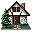Home Existing users log in here or new users sign up here. Kinematics Puzzles Mechanics Puzzles Electric Circuits Puzzles Magnetism Puzzles Kinematics Surprising Data Mechanics Surprising Data Electrostatics Surprising Data Fluids Surprising Data Optics Surprising Data

### Finding the coefficient of friction: Application Experiment

#### Aim

Determine the coefficient of kinetic friction between the tissue box and the table.

#### Prior Knowledge

• Conservation of momentum
• Transformation of energy
• Kinetic energy, gravitational potential energy, internal energy, work
• Friction force, static friction force, kinetic friction force
Description of the Experiment

Observe the two experiments below. Use each to determine the coefficient of kinetic friction between the tissue box and the table. List all of the assumptions that you made. Describe how you will use the video to determine the necessary quantities. List all physics explanations/relationships you will use to determine the coefficient of friction. Describe the mathematical procedure that you will use to find the coefficient of kinetic friction from the measured physical quantities. Decide whether you have a reasonable agreement between the results of the two experiments.

Carefully examine the assumptions that you use.

The flour-filled balloon has a mass of 54.7g, the tissue-box has a mass of 161.1g.